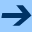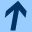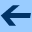Arrays and two-dimensional arrays

# Arrays and two-dimensional arrays

Arrays are the most fundamental data type to store many elements, and are implemented directly by the virtual machine (by contrast, (mutable) lists are implemented in a library, using an array to store the elements).

You can think of an array as a mutable list of fixed size—that is, you cannot add or remove elements. Once created, the size of an array stays the same.

Like lists, arrays can be created by listing the elements:

>>> val a = arrayOf(1, 2, 3, 4, 5)

They support most of the methods available for mutable lists:
>>> a.size
5
>>> a = 99
>>> a
4
>>> a.joinToString()
99, 2, 3, 4, 5
>>> println(a)
[Ljava.lang.Integer;@16d48c9

Note that printing an array directly does not list the elements nicely as happens for List or MutableList.

When you want to create a large array, or an array whose size you have somehow computed, you cannot list the initial elements. Instead, you provide the number of elements, and a piece of code that computes the value of the element. This piece of code can make use of the magic variable it which gives the index of the element:

>>> val a = Array(10) { 0 }
>>> a.joinToString()
0, 0, 0, 0, 0, 0, 0, 0, 0, 0
>>> val b = Array(13) { it * it }
>>> b.joinToString()
0, 1, 4, 9, 16, 25, 36, 49, 64, 81, 100, 121, 144
>>> val s = Array(8) { "" }
>>> s.joinToString()
, , , , , , ,


There is usually no good reason to use an array instead of a (mutable) list. However, the args variable that provides the command line arguments to a Kotlin script, is actually an array, of type Array<String>.

One important thing to remember is that the equality operators do not check the contents of arrays. They will only tell you if two variables are reference for the same array. This is different from the behaviour of the equality operator for lists (or in fact, any other standard collection type):

>>> val a = listOf(1, 2, 3, 4)
>>> val b = listOf(1, 2, 3, 4)
>>> a == b
true
>>> val d = arrayOf(1, 2, 3, 4)
>>> val e = arrayOf(1, 2, 3, 4)
>>> d == e
false
>>> val f = d
>>> f == d
true
>>> f == e
false


#### Two-dimensional arrays

Another opportunity to use arrays is when you need a two-dimensional storage area, for instance to represent a board in a game. A two-dimensional array uses two indices, often called row and column, to acces $$m \times n$$ elements. It is implemented by making one array for each row (these arrays are indexed by the column number), and then one array (indexed by the row number) to store the rows. (One could do the same with lists, but that would waste quite a bit of storage.)

The following example creates a two-dimensional array with 5 rows and 8 columns, filled with zeroes:

>>> val b = Array(5) { Array(8) { 0 } }

The type of b is Array<Array<Int>>. We access its elements using row and column number:
>>> b = 9
>>> b = 13


Since b itself is the array storing the rows, we can get the number of rows as b.size. The number of columns is the length of each element of b, for instance b.size:

>>> b.size
5
>>> b.size
8


You will need to write a function to display the board nicely. For debugging, something like the following will be good enough:

>>> b.joinToString(separator="\n", transform={ it.joinToString() } )
0, 0, 0, 0, 0, 13, 0, 0
0, 0, 0, 0, 0, 0, 0, 0
0, 0, 0, 0, 0, 0, 0, 9
0, 0, 0, 0, 0, 0, 0, 0
0, 0, 0, 0, 0, 0, 0, 0Arrays and two-dimensional arrays